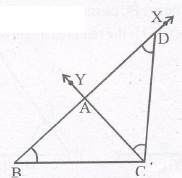## Maths Class 9 Notes for Constructions

INTRODUCTION

Geometrical construction is the process of drawing a geometrical figure using only two instruments- an ungraduated ruler, also called a straight edge and a compass.

BASIC CONSTRUCTIONS

Statement 1: To construct the bisector of a given angle.

Given : An angle PQR,

Required : construct its bisector.

Steps of Construction:

1. By Taking Q as centre and any radius, draw an arc which intersect the rays QP and QR, at E and D respectively (see fig (i))

2. Next By taking D and E as centres and with the radius > 1/2 DE draw arcs which intersect each other, at F.

3. Draw the ray QF (see fig(ii)) is the required bisector of the angle PQR.Statement 2: To construct the perpendicular bisector of a given line segment.

Given : A line segment AB

Required : construct its perpendicular bisector.

Steps of Construction :

1. Taking A and B as centres and radius more than 1/2 AB draw arcs on both sides of the line segment AB (to intersect each other).

2. Let these arcs intersect each other at P and Q. Join PQ (see fig)

3. Let PQ intersect AB at the point M. Then line PMQ is the required perpendicular bisector of AB.Statement 3: To construct an angle of 60° at the initial point of a given ray.

Given : A ray PQ with initial point P

Required : To construct a ray PC such that ∠CPQ = 60° .

Steps of Construction :

1. Taking P as centre and any radius, draw an arc, which intersects PQ, say at a point D.

2. Now, Taking D as centre and with the same radius as in step 1., draw an arc which is intersecting the previously drawn arc, at a point

3. Draw the ray PC passing through E. (see fig (ii))

Then ∠CPQ is the required angle of 60°.SOME CONSTRUCTIONS OF TRIANGLES

1. Rules of Congruency of Two Triangles

(i) SAS : Two triangles are congruent, if any two sides and the included angle of one triangle are equal to any two sides and the included angle of the other triangle.

(ii) SSS : Two triangles are congruent if the three sides of one triangle are equal to the three sides of the other triangle.

(iii) ASA : Two triangles are congruent if any two angles and the included side of one triangle are equal to the two angles and the included side of the other triangle.

(iv) RHS : Two right triangles are congruent if the hypotenuse and a side of one triangle are respectively equal to the hypotenuse and a side of the other triangle.

2. Uniqueness of a Triangle

A triangle is unique if

(i) two sides and the included angle is given

(ii) three sides and angle is given

(iii) two angles and the included side is given and,

(iv) in a right triangle, hypotenuse and one side is given.

Note : At least three parts of a triangle have to be given for constructing it but not all combinations of three parts are sufficient for the purpose.

BASIC CONSTRUCTIONS OF TRIANGLES:

Statement 1: To construct a triangle, given its base, a base angle and sum of other two sides.

Given : Base BC, a base angle, say ∠B and the sum AB + AC of the other two sides of a triangle ΔABC

Required: To construct a A ABC.

Steps of Construction1. Draw the base BC and at the point B make an angle, say XBC equal to the given angle.

2. Cut a line segment BD equal to AB + AC from the ray BX.

3. Join DC and make an angle DCY equal to L BDC.

4. Let CY intersect BX at A (see fig.)

Then, ABC is the required triangle.

Note : The construction of the triangle is not possible if the sum AB + AC ≤ BC.

Statement 2: To construct a triangle given its base, a base angle and the difference of the other two sides.

Given : The base BC, a base angle, say Z B and the difference of other two sides AB — AC or AC — AB.

Require : Construct the triangle ABC.

Case (i) : Let AB > AC that is AB —AC is given.

Steps of Construction :1. Draw the base BC and at point B make an angle say XBC equal to the given angle.

2. Cut the line segment BD equal to AB — AC from ray BX.

3. Join DC and draw the perpendicular bisector, say PQ of DC.

4. Let it intersect BX at a point A. Join AC (see fig.)

I hen ABC is the required triangle.

Case (ii) : Let AB < AC that is AC — AB is given.

Steps of Construction :1. Draw the base BC and at B make an angle XBC equal to the given angle.

2. Cut the line segment BD equal to AC — AB from the line BX extended on opposite side of line segment BC.

3. Join DC and draw the perpendicular bisector, say PQ of DC.

4. Let PQ intersect BX at A. Join AC (see fig.) Then, ABC is the required triangle.

Statement 3: To construct a triangle, given its perimeter and its two base angles.

Given : The base angles, say ∠B and ∠C and BC + CA + AB.

Required : construct the triangle ABC.

Steps of Construction :

1. Draw a line segment, say XY equal to BC + CA -FAB.

2. Make angles LXY equal to ∠B and MYX equal to∠C.

3. Bisect ∠LXY and ∠MYX. Let these bisectors intersect at a point A . (see fig (i))4. Draw perpendicular bisectors PQ of AX and RS of AY.

5. Let PQ intersect XY at B and RS intersect XY at C. Join AB and AC. (see fig (ii))Then ABC is the required triangle.